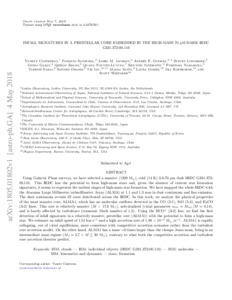# Infall Signatures in a Prestellar Core embedded in the High-Mass 70 $μ$m Dark IRDC G331.372-00.116

Contreras, Y, Sanhueza, P, Jackson, JM, Guzman, AE, Longmore, SN, Garay, G, Zhang, Q, Nguyen-Luong, Q, Tatematsu, K, Nakamura, F, Sakai, T, Ohashi, S, Liu, T, Saito, M, Gomez, L, Rathborne, J and Whitaker, S (2018) Infall Signatures in a Prestellar Core embedded in the High-Mass 70 $μ$m Dark IRDC G331.372-00.116. Astrophysical Journal, 861 (1). ISSN 0004-637XPreview
Text
Infall Signatures in a Prestellar Core embedded in the High-Mass 70 $μ$m Dark IRDC G331.372-00.116.pdf - Accepted Version

Using Galactic Plane surveys, we have selected a massive (1200 M$_\odot$), cold (14 K) 3.6-70 $\mu$m dark IRDC G331.372-00.116. This IRDC has the potential to form high-mass stars and, given the absence of current star formation signatures, it seems to represent the earliest stages of high-mass star formation. We have mapped the whole IRDC with the Atacama Large Millimeter/submillimeter Array (ALMA) at 1.1 and 1.3 mm in dust continuum and line emission. The dust continuum reveals 22 cores distributed across the IRDC. In this work, we analyze the physical properties of the most massive core, ALMA1, which has no molecular outflows detected in the CO (2-1), SiO (5-4), and H$_2$CO (3-2) lines. This core is relatively massive ($M$ = 17.6 M$_\odot$), subvirialized (virial parameter $\alpha_{vir}=M_{vir}/M=0.14$), and is barely affected by turbulence (transonic Mach number of 1.2). Using the HCO$^+$ (3-2) line, we find the first detection of infall signatures in a relatively massive, prestellar core (ALMA1) with the potential to form a high-mass star. We estimate an infall speed of 1.54 km s$^{-1}$ and a high accretion rate of 1.96 $\times$ 10$^{-3}$ M$_\odot$ yr$^{-1}$. ALMA1 is rapidly collapsing, out of virial equilibrium, more consistent with competitive accretion scenarios rather than the turbulent core accretion model. On the other hand, ALMA1 has a mass $\sim$6 times larger than the clumps Jeans mass, being in an intermediate mass regime ($M_{J}=2.7<M\lesssim$ 30 M$_\odot$), contrary to what both the competitive accretion and turbulent core accretion theories predict.View Item